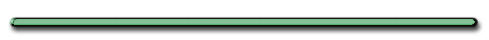HomeEver see an object roll UP hill?

Video Instruction

showmethephysics.com

C. Solving Word Problemsex) A car accelerates uniformly from rest at a rate of +10 m/s2. How far will the car travel in 4 seconds?

• Step 1 - Read and underline all the important information

ex) A car accelerates uniformly from rest at a rate of +10 m/s2. How far will the car travel in 4 seconds?

Step 2 - Turn all your information into letters

Vi = 0 (starts from rest)

a = +10 m/s2

t = 4 seconds

d = ?

Step 3 - Substitute and Solve Equation:Δd = Vit + (1/2)aΔt2

= 0 m/s(4 sec)+1/2(10 m/s2)(4s)2

Δd = 0 + 5(16)

Δd = 80 mD. Deriving the 2nd displacement equation:

`Vf2 = Vi2 + 2aΔd`
` `
` `
`d = VΔt`
` `
` `
` `
 Δd = (Vf + Vi) Δt 2
`	`
` `
` `
` `
` `
` `
` `
` `
``` since Vf = Vi + aΔt
then Δt = (Vf -Vi)/a```
` `
` `
` `
` `
` `
` `
 Δd = (Vf + Vi) (Vf - Vi) 2 a
` `
 Δd = (Vf2 - Vi2) 2a
`Cross Multiply`
` `
` `
` `
` `
` `
`	`
 2aΔd = Vf2 - Vi2
` `
 Vf2 =(Vi2 + 2aΔd)Ex) A car with an initial velocity of +6 m/s accelerates at a rate 2 m/s2. Find the cars final velocity after it travels 7 m from its initial position"Step 1 - Read and underline all the  important information "

Ex) A car with an initial velocity of +6 m/s accelerates at a rate 2 m/s2. Find the cars final velocity after it travels 7 m from its initial position"Step 2 - Turn all your information  into letters"

Vi = 6 m/s
a = 2 m/s2
Vf = ?
d = 7 meters"Step 3 - Substitute and Solve"Vf2 = Vi2 + 2aΔd

Vf2  = (6 m/s)2 + 2(2 m/s2)(7 m)

Vf2  = 36 + 4(7)

Vf2 = 64

Vf = 8 m/sDisplacement Plots